SSC  >  SSC CPO Mock Test (Quant Apt) 9

# SSC CPO Mock Test (Quant Apt) 9

Test Description

## 50 Questions MCQ Test SSC CPO & Constable - Mock Tests & Previous Year Papers | SSC CPO Mock Test (Quant Apt) 9

SSC CPO Mock Test (Quant Apt) 9 for SSC 2023 is part of SSC CPO & Constable - Mock Tests & Previous Year Papers preparation. The SSC CPO Mock Test (Quant Apt) 9 questions and answers have been prepared according to the SSC exam syllabus.The SSC CPO Mock Test (Quant Apt) 9 MCQs are made for SSC 2023 Exam. Find important definitions, questions, notes, meanings, examples, exercises, MCQs and online tests for SSC CPO Mock Test (Quant Apt) 9 below.
Solutions of SSC CPO Mock Test (Quant Apt) 9 questions in English are available as part of our SSC CPO & Constable - Mock Tests & Previous Year Papers for SSC & SSC CPO Mock Test (Quant Apt) 9 solutions in Hindi for SSC CPO & Constable - Mock Tests & Previous Year Papers course. Download more important topics, notes, lectures and mock test series for SSC Exam by signing up for free. Attempt SSC CPO Mock Test (Quant Apt) 9 | 50 questions in 30 minutes | Mock test for SSC preparation | Free important questions MCQ to study SSC CPO & Constable - Mock Tests & Previous Year Papers for SSC Exam | Download free PDF with solutions
 1 Crore+ students have signed up on EduRev. Have you?
SSC CPO Mock Test (Quant Apt) 9 - Question 1

### The present ages of 3 persons are in the proportion of 4:7:9. Eight yrs ago, the sum of their ages was 56. Find their present ages.

SSC CPO Mock Test (Quant Apt) 9 - Question 2

### For Rs 300 you can buy either packet-1 containing 9 paper weight and 7 ball pens or pacjet-II containing 6 paper weight and 13 ball pens. The price of each paper weight is

Detailed Solution for SSC CPO Mock Test (Quant Apt) 9 - Question 2 Let Price of each paper weight be Rs. x and ball Pens be Rs. y
9x + 7y = 300 .....(i)
6x + 13y = 300 .....(ii)
Solving (i) & (ii) we get
y = 12 & x = 24
SSC CPO Mock Test (Quant Apt) 9 - Question 3

### If one root of the quadratic equation 3x2 - 10x + p = 0 is 1 3 , then the value of p and other root respectively are

Detailed Solution for SSC CPO Mock Test (Quant Apt) 9 - Question 3

Let 1/3 , α be the roots of the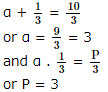SSC CPO Mock Test (Quant Apt) 9 - Question 4
The square root of (2722-1282) is
SSC CPO Mock Test (Quant Apt) 9 - Question 5

x2 varies directly as y3 and when x=6,y=3.Which of the following equation correctly represents the relationship between x and y?

Detailed Solution for SSC CPO Mock Test (Quant Apt) 9 - Question 5

x2 ∝ y3
⇒ x2 = Ky3
or, x = 6, y = 3
⇒ K = 4/3
So, 3x2 = 4y3

SSC CPO Mock Test (Quant Apt) 9 - Question 6
The average of marks obtained by 120 candidates in a certain examination is 35. If the average marks obtained by passed candidates is 39 and that of the failed candidates is 15, what is the number of candidates who passed the examination?
SSC CPO Mock Test (Quant Apt) 9 - Question 7

The area of the circumcircle of the equilateral triangle whose one side lies on the diagonal of a square with side 4 cm is (use π = 3.14)

SSC CPO Mock Test (Quant Apt) 9 - Question 8

Size of a tile is 9 inches by 9 inches. The number of tiles needed to cover a floor of 12 feet by 12 feet is

Detailed Solution for SSC CPO Mock Test (Quant Apt) 9 - Question 8

Length of floor = 12 feet
Breath of floor = 12 feet
So Area of floor = 12 × 12 = 144 feet
Area of tile = 9/12 × 9/12 = 9/16 feet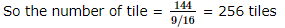SSC CPO Mock Test (Quant Apt) 9 - Question 9

Area of a square field is 1 hectare. The length of another square field is 1% more than the length of this square field. The difference in their areas is

Detailed Solution for SSC CPO Mock Test (Quant Apt) 9 - Question 9

1 hectare = 10000 m2
Area of a square field = 10000 m2
let length of a square field be x m
then x2 = 10000
x = 100m
length of other square field = 100 + 100 x 1/100 = 101
Area of Another square field = (101)2 = 10201 m2
∴ Difference in their areas = 10201 - 10000
= 201 m2

SSC CPO Mock Test (Quant Apt) 9 - Question 10

The average of three numbers is 135. The largest number is 180 and the difference of the others is 25. The smallest number is

Detailed Solution for SSC CPO Mock Test (Quant Apt) 9 - Question 10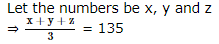⇒ x + y + z = 405
But let x = largest number i.e. 180
⇒ y + z = 225 .....(1)
Also, y - z = 25 .....(2)
Solving (1) and (2)
We get 2y = 250
⇒ y = 125
⇒ z = 125 - 25 = 100
Hence, the smallest number among the three numbers is 100

SSC CPO Mock Test (Quant Apt) 9 - Question 11
The equation of the straight line which is perpendicular to y = x and passes through (3, 2) is :
Detailed Solution for SSC CPO Mock Test (Quant Apt) 9 - Question 11 y = x
⇒ x - y = 0
⇒ y + x + λ = 0
⇒ 2 + 3 + λ = 0
⇒ λ = -5
⇒ x + y = 5
SSC CPO Mock Test (Quant Apt) 9 - Question 12
How much time will it take for an amount of Rs. 450 to yield Rs. 81 as interest at 4.5% per annum of simple interest?
SSC CPO Mock Test (Quant Apt) 9 - Question 13
Which of the following is a perfect square as well as a cube? 343,125,81 or 64
SSC CPO Mock Test (Quant Apt) 9 - Question 14

The value of [(0.051×0.051×0.051+0.041×0.041×0.041)/(0.051×0.051-0.051×0.041+0.041×0.041)] is :

SSC CPO Mock Test (Quant Apt) 9 - Question 15
A specific type of cloth costs Rs 50 a metre and a buyer gets 8% discount on the total cost,if he buys 125 metres or more. The cost of 625 metres of cloth is
SSC CPO Mock Test (Quant Apt) 9 - Question 16
Diagonals AC and BD of || gm ABCD meet at O. If AP = QC then quadrilateral PBQD is a
Detailed Solution for SSC CPO Mock Test (Quant Apt) 9 - Question 16 DO = OB and AO = OC. Also AP = CQ
$⇒$ AO - AP = OC - CQ
$⇒$ OP = OQ
Hence, PBQD is a || gm.
SSC CPO Mock Test (Quant Apt) 9 - Question 17

In the given figure AD bisects ΔBAC. If AB = 10 cm, AC = 6 cm and BD = 12cm, then length of CD is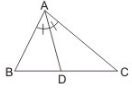Detailed Solution for SSC CPO Mock Test (Quant Apt) 9 - Question 17

By internal angle bisector theorem, the bisector of vertical angle of triangle divides the base in the ratio of the other two sides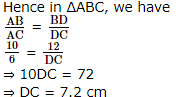SSC CPO Mock Test (Quant Apt) 9 - Question 18
If the two angles of a triangle are equal to the corresponding angles of another triangle, then the triangles are called :
SSC CPO Mock Test (Quant Apt) 9 - Question 19
(1095/1168) when expressed in simplest form is :
SSC CPO Mock Test (Quant Apt) 9 - Question 20
A pole is standing erect on the horizontal ground.The tip of the pole is tied tight with a rope of length √12 at a point on the ground. If the rope is making an angle of 30� with the horizontal,then the height of the pole is
SSC CPO Mock Test (Quant Apt) 9 - Question 21
The correct statement out of the following is

Detailed Solution for SSC CPO Mock Test (Quant Apt) 9 - Question 21 ∠A = ∠F; ∠B = ∠E and ∠C = ∠D
Hence, ∆ABC$\sim$∆FED.
SSC CPO Mock Test (Quant Apt) 9 - Question 22
Two numbers are in the ratio 5:4 and there difference is 10.What is the largest number?
SSC CPO Mock Test (Quant Apt) 9 - Question 23

What percentage of numbers from 1 to 70 have squares that end in the digit 1?

Detailed Solution for SSC CPO Mock Test (Quant Apt) 9 - Question 23

The numbers which have 1 or 9 in the unit's digit, have squares that end in the digit 1.
Such numbers from 1 to 70 are 1, 9, 11, 19, 21, 29, 31, 39, 41, 49, 51, 59, 61, 69
Number of such number = 14
∴ Required percentage = (14/70 × 100)% = 20%

SSC CPO Mock Test (Quant Apt) 9 - Question 24
A district has 64000 inhabitants. If the population increases at the rate of 2(1/2)% per annum, then the number of inhabitants at the end of 3 years will be
SSC CPO Mock Test (Quant Apt) 9 - Question 25
A tap can fill a tank in 6 hrs. After half the tank is filled, three more similar taps are opened. What is the total time taken to fill the tank completely?
SSC CPO Mock Test (Quant Apt) 9 - Question 26

A man gains 20% by selling an article for a certain price. If he sells it at double the price, the percentage of profit will be:

Detailed Solution for SSC CPO Mock Test (Quant Apt) 9 - Question 26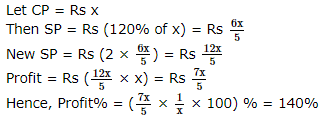SSC CPO Mock Test (Quant Apt) 9 - Question 27

A man purchased a box full of pencils at the rate of 7 for Rs 9 and sold all of them at the rate of 8 for Rs 11. In this transaction, he gained Rs. 10. How many pencils did the box contain?

Detailed Solution for SSC CPO Mock Test (Quant Apt) 9 - Question 27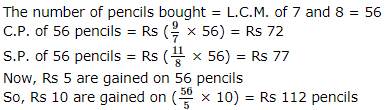SSC CPO Mock Test (Quant Apt) 9 - Question 28

In Δ A B C ,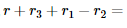SSC CPO Mock Test (Quant Apt) 9 - Question 29

Rs 6400 are divided among three workers in the ratio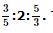The share of the second worker (in rupees)is

Detailed Solution for SSC CPO Mock Test (Quant Apt) 9 - Question 29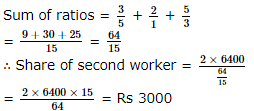SSC CPO Mock Test (Quant Apt) 9 - Question 30
In a factory,the ratio of male to female workers was 5:3. If the number of female workers was less by 40,then what was the total number of workers in the factory?
SSC CPO Mock Test (Quant Apt) 9 - Question 31

The two geometric means between 1 and 64 are

Detailed Solution for SSC CPO Mock Test (Quant Apt) 9 - Question 31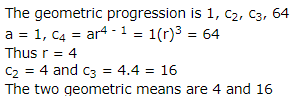SSC CPO Mock Test (Quant Apt) 9 - Question 32
A sum of Rs 600 amounts to Rs 720 in 4 yrs. What will it amount to if the rate of interest is increased by 2%?
SSC CPO Mock Test (Quant Apt) 9 - Question 33
In a garden, there are n rows and n columns with a tree in each cell. If the total number of trees is 225 in the garden, then the value of n is
SSC CPO Mock Test (Quant Apt) 9 - Question 34

A gun is fired at a distance of 3.32 km from Barbar. He hears the sound 10 sec later. Find the speed of the sound.

Detailed Solution for SSC CPO Mock Test (Quant Apt) 9 - Question 34

Distance = 3.32 × 1000 = 3320 m
Time = 10s
∴ Speed = 3320/10 = 332 m/s

SSC CPO Mock Test (Quant Apt) 9 - Question 35

If 4 workers can make 42 toys in 6 days, how many toys can 12 workers make in 3 days?

Detailed Solution for SSC CPO Mock Test (Quant Apt) 9 - Question 35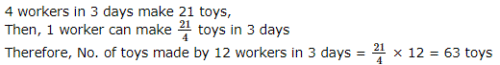SSC CPO Mock Test (Quant Apt) 9 - Question 36
If cosec A + cot A = 11/2, then tan A is
SSC CPO Mock Test (Quant Apt) 9 - Question 37

Maximum value of cos²x+cos²y-cos²z is

SSC CPO Mock Test (Quant Apt) 9 - Question 38
The length of an edge of a hollow cube open at one face is √3 metres. What is the length of the largest pole that it can accomodate?
SSC CPO Mock Test (Quant Apt) 9 - Question 39

What is the volume of a cube (in cubic cm) whose diagonal measures 4√3 cm?

Detailed Solution for SSC CPO Mock Test (Quant Apt) 9 - Question 39

Length of the diagonal of the cube = 4√3 cm
So side = 4cm
Volume = (4)3 = 64 cubic cm

SSC CPO Mock Test (Quant Apt) 9 - Question 40

The greatest value of 3 cos θ + 4 sin θ is :

Detailed Solution for SSC CPO Mock Test (Quant Apt) 9 - Question 40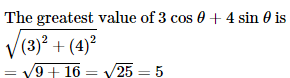SSC CPO Mock Test (Quant Apt) 9 - Question 41
The volumes of two cubes are in the ratio 8:27. The ratio of their surface areas is :
SSC CPO Mock Test (Quant Apt) 9 - Question 42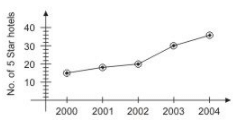The above graph shows the number of 5 Star Hotels in Delhi from 2000 to 2004. Observe the above line graph and answer the following question:

Q. Percentage increase in the number of 5-Star Hotels in 2004 as compared to 2000 is

Detailed Solution for SSC CPO Mock Test (Quant Apt) 9 - Question 42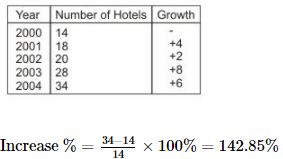SSC CPO Mock Test (Quant Apt) 9 - Question 43The above graph shows the number of 5 Star Hotels in Delhi from 2000 to 2004. Observe the above line graph and answer the following question:

Q. What percentage of number of 5-star hotels in 2000 is the number in 2004?

Detailed Solution for SSC CPO Mock Test (Quant Apt) 9 - Question 43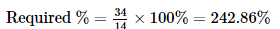SSC CPO Mock Test (Quant Apt) 9 - Question 44The above graph shows the number of 5 Star Hotels in Delhi from 2000 to 2004. Observe the above line graph and answer the following question:

Q. The ratio of number of 5-star hotels in 2001 to the number of 5-star hotels in 2003 is:

Detailed Solution for SSC CPO Mock Test (Quant Apt) 9 - Question 44

Required ratio = 18 : 28 = 9 : 14

SSC CPO Mock Test (Quant Apt) 9 - Question 45The above graph shows the number of 5 Star Hotels in Delhi from 2000 to 2004. Observe the above line graph and answer the following question:

Q. The year in which growth as compared to the previous year is maximum in

Detailed Solution for SSC CPO Mock Test (Quant Apt) 9 - Question 45

Clearly maximum growth is in 2003 (+8)

SSC CPO Mock Test (Quant Apt) 9 - Question 46

Percentage of students in various courses(A,B,C,D,E,F) and Percentage of girls out of these.
Total Students : 1200
(800 girls + 400 boys)
Percentage in various courses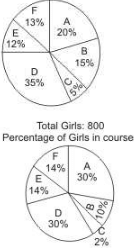Q.  For which course is the number of boys the minimum ?

Detailed Solution for SSC CPO Mock Test (Quant Apt) 9 - Question 46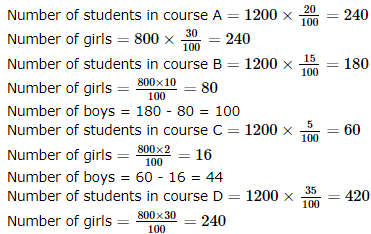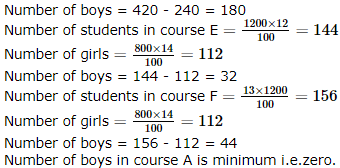SSC CPO Mock Test (Quant Apt) 9 - Question 47

Percentage of students in various courses(A,B,C,D,E,F) and Percentage of girls out of these.
Total Students : 1200
(800 girls + 400 boys)
Percentage in various coursesQ.  How many girls are there in course C ?

Detailed Solution for SSC CPO Mock Test (Quant Apt) 9 - Question 47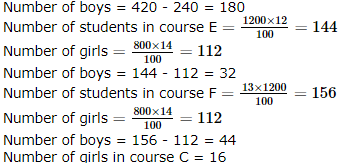SSC CPO Mock Test (Quant Apt) 9 - Question 48

Percentage of students in various courses(A,B,C,D,E,F) and Percentage of girls out of these.
Total Students : 1200
(800 girls + 400 boys)
Percentage in various coursesQ. For course D what is the respective ratio of boys and girls ?

Detailed Solution for SSC CPO Mock Test (Quant Apt) 9 - Question 48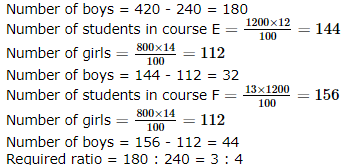SSC CPO Mock Test (Quant Apt) 9 - Question 49

Percentage of students in various courses(A,B,C,D,E,F) and Percentage of girls out of these.
Total Students : 1200
(800 girls + 400 boys)
Percentage in various coursesQ. For which pair of courses is the number of boys the same ?

Detailed Solution for SSC CPO Mock Test (Quant Apt) 9 - Question 49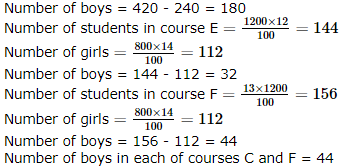SSC CPO Mock Test (Quant Apt) 9 - Question 50

Percentage of students in various courses(A,B,C,D,E,F) and Percentage of girls out of these.
Total Students : 1200
(800 girls + 400 boys)
Percentage in various coursesQ. For course E, the number of girls is how much percent more than the boys for course E ?

Detailed Solution for SSC CPO Mock Test (Quant Apt) 9 - Question 50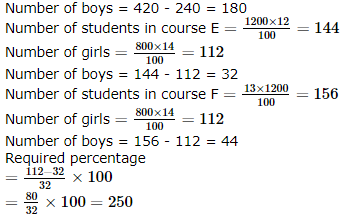## SSC CPO & Constable - Mock Tests & Previous Year Papers

1 docs|63 tests
 Use Code STAYHOME200 and get INR 200 additional OFF Use Coupon Code
Information about SSC CPO Mock Test (Quant Apt) 9 Page
In this test you can find the Exam questions for SSC CPO Mock Test (Quant Apt) 9 solved & explained in the simplest way possible. Besides giving Questions and answers for SSC CPO Mock Test (Quant Apt) 9, EduRev gives you an ample number of Online tests for practice

1 docs|63 tests